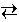# Chemical Equilibria

## Equilibrium Constant

For the hypothetical chemical reaction

a A + b Bc C + d D

the equilibrium constant is defined as

KC   =
[C]c [D]d [A]a [B]b

The notation [A] signifies the molar concentration of species A. An alternative expression for the equilibrium constant involves partial pressures.

KP   =
PC c PD d PA a PB b

Note that the expression for the equilibrium constant includes only solutes and gases. Pure solids and liquids do not appear in the expression. For example, in the reaction below, the gas-phase species H2O and H2 appear in the equilibrium expression but the solids CaH2 and Ca(OH)2 do not appear.

CaH2 (s) + 2 H2O (g)Ca(OH)2 (s) + 2 H2 (g)

KC   =
[H2]2 [H2O]2

The equilibrium constant is most readily determined by allowing a reaction to reach equilibrium, measuring the concentrations of the various solution-phase or gas-phase reactants and products, and substituting these values into the Law of Mass Action.

## Experiment: Part 1

### Objective:

Determine the equilibrium constant for a chemical reaction.

In this part of the experiment, the following reaction is studied.

NaHCO3 (s)NaOH (s) + CO2 (g)

The steps in the experiment are those that would be performed in practice in the laboratory:

1. A 1 gram portion of sodium hydrogen carbonate powder is placed in a 500. mL container.
2. The container is evacuated with a vacuum pump to remove all gases.
3. The container is heated to 800. K and the reaction is allowed to reach equilibrium.
4. The equilibrium pressure of the carbon dioxide gas is measured with a manometer.

At room temperature, the reaction does not proceed at a measurable rate. At 800. K, however, the rate of reaction is relatively fast and equilibrium is readily achieved.

A manometer is attached to the container to measure the pressure of carbon dioxide in the bulb. (In this experiment, carbon dioxide is the only gas in the container, so the pressure is also the partial pressure of CO2.) Assume the sodium hydrogen carbonate occupies a negligible fraction of the volume of the 500. mL bulb. Also assume that the connection to the manometer have a negligible volume. The stopcock to the bulb is always open, because the bulb is always open to the manometer so that the pressure may be continually monitored. Carbon dioxide is a colorless gas.

Use the experimental data to calculate KP and KC for this reaction. In this part of the experiment, the value KP and KC are provided to permit you to check your work.

The gas constant is R = 0.08206 L atm K-1 mole-1.

Reset Experiment

Evacuate Bulb

Heat System

Show KP

Show KC

## Experiment: Part 2

This part of the experiment is identical to Part 1 except the values of KP and KC are not provided and the reaction under investigation is

Ca(OH)2 (s)CaO (s) + H2O (g)

The steps in the experiment are the same as those in Part 1. In this case, the equilibrium is studied at 700. K. As before, the volume of the container is 500. mL.

The gas constant is R = 0.08206 L atm K-1 mole-1.

Reset Experiment